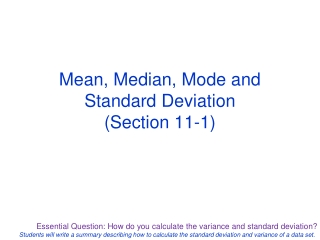DownloadDownload PresentationMean, Median, Mode and Standard Deviation (Section 11-1)

# Mean, Median, Mode and Standard Deviation (Section 11-1)

Télécharger la présentation## Mean, Median, Mode and Standard Deviation (Section 11-1)

- - - - - - - - - - - - - - - - - - - - - - - - - - - E N D - - - - - - - - - - - - - - - - - - - - - - - - - - -
##### Presentation Transcript

1. Mean, Median, Mode and Standard Deviation(Section 11-1)

2. Statistics: Numerical values used to summarize and compare sets of data Measure of central tendency: A number used to represent the center or middle of a set of data values.

3. Example1 Below are the intervals (in minutes) between eruptions of the Old Faithful geyser in Yellowstone National Park. Calculate the mean, median, and mode for the dataset: 98 92 95 87 96 90 65 92 95 93 98 94

4. Measure of dispersion: A statistic that tells you how dispersed, or spread out, data values are Range: The difference between the greatest and least data values.

5. Standard deviation: A measure that describes the typical difference (or deviation) between a data value and the mean Variance: The average (mean) of the squared differences from the Mean

6. HW #____ Practice WS 11-1